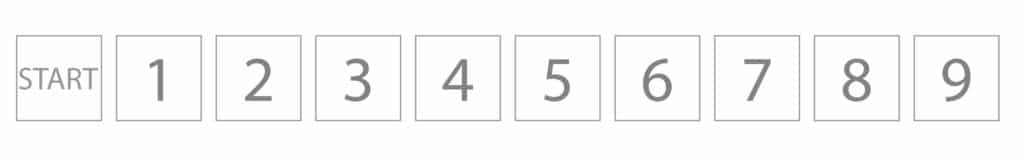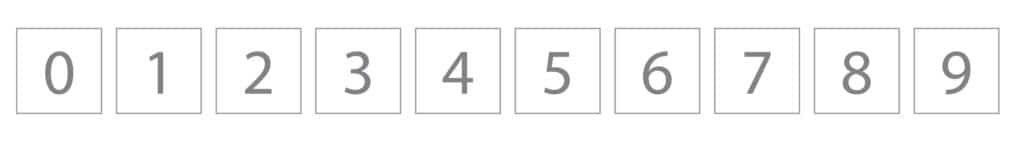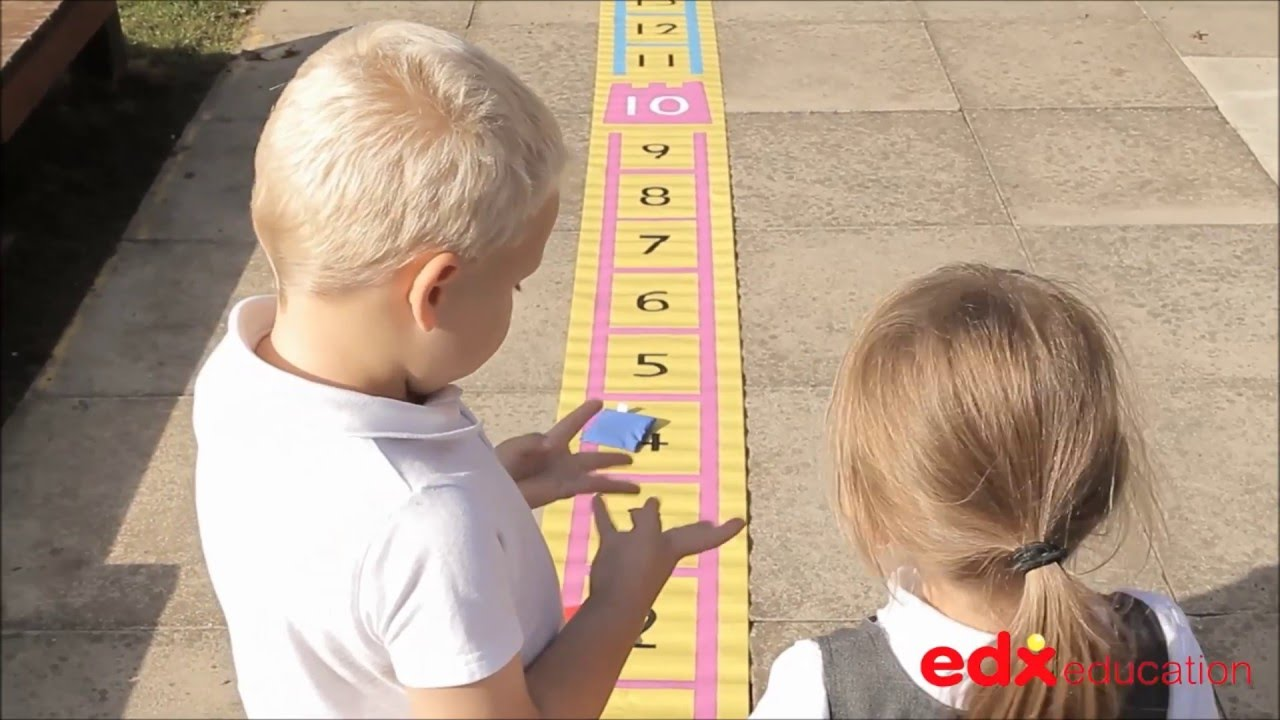# Number Tracks

#### • Number: Counting, Counting On, Counting Back, Addition, Subtraction

Number tracks are made up of a number of cells, which are generally square or rectangular. Each cell is numbered as students are counting cells. This helps to explain why number tracks start at one. Game tracks will often include a cell labeled ‘start’, where the counters or pawns are placed prior to beginning the game.Other number tracks are numbered from zero.### Number Tracks Videos### Mathematical Language

Addition, subtraction, counting, number names 1, 2, 3, count on, count back, greater than, less than.

### Using Number Tracks

When working with young children:
• Point to a number on the track. Ask the students to tell you the number that comes before or after the number.
• Point to a number on the track and ask the students to identify the number that is one less or one more than the number.
• Point to a number. Ask the students to state a number that is greater than or less than the number.
• Point to two numbers. Ask the students to identify which number is greater or less than the other number.
• State two numbers eg 4 and 6 and ask the students to identify the number that comes between the two numbers.
• Practise counting forward or backwards in ones from any number on the track.

To help children progress from a number track to a number line they can use a counter number line.

Children will begin by placing counters onto the strip and then write onto the number line. Likewise links may be made to a number track. Later
students will transition to number lines, using a Counter Number Line or pegging numbers onto a line.